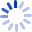# Principles of Digital Image Processing: Core Algorithms

This textbook is the second of three volumes providing a modern, algorithmic introduction to digital image processing. It presents a critical selection of algorithms, illustrated explanations and concise mathematical derivations.• Book Details
• Seller